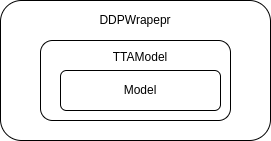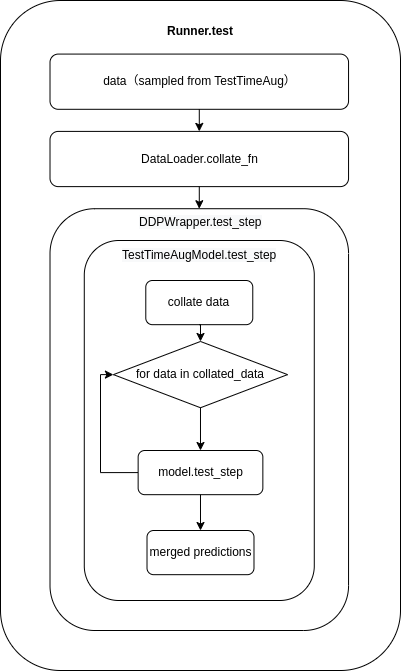Shortcuts

# Test time augmentation¶

Test time augmentation (TTA) is a data augmentation strategy used during the testing phase. It involves applying various augmentations, such as flipping and scaling, to the same image and then merging the predictions of each augmented image to produce a more accurate prediction. To make it easier for users to use TTA, MMEngine provides BaseTTAModel class, which allows users to implement different TTA strategies by simply extending the BaseTTAModel class according to their needs.

The core implementation of TTA is usually divided into two parts:

1. Data augmentation: This part is implemented in MMCV, see the api docs TestTimeAug for more information.

2. Merge the predictions: The subclasses of BaseTTAModel will merge the predictions of enhanced data in the test_step method to improve the accuracy of predictions.

## Get started¶

A simple example of TTA is given in examples/test_time_augmentation.py

### Prepare test time augmentation pipeline¶

BaseTTAModel needs to be used with TestTimeAug implemented in MMCV:

tta_pipeline = [
dict(
type='TestTimeAug',
transforms=[
[dict(type='Resize', img_scale=(1333, 800), keep_ratio=True)],
[dict(type='RandomFlip', flip_ratio=0.),
dict(type='RandomFlip', flip_ratio=1.)],
[dict(type='PackXXXInputs', keys=['img'])],
])
]


The above data augmentation pipeline will first perform a scaling enhancement on the image, followed by 2 flipping enhancements (flipping and not flipping). Finally, the image is packaged into the final result using PackXXXInputs.

### Define the merge strategy¶

Commonly, users only need to inherit BaseTTAModel and override the BaseTTAModel.merge_preds to merge the predictions of enhanced data. merge_preds accepts a list of enhanced batch data, and each element of the list means the enhanced single data of the batch.

The BaseTTAModel class requires inferencing on both flipped and unflipped images and then merges the results. The merge_preds method accepts a list where each element represents the results of applying data augmentation to a single element of the batch. For example, if batch_size is 3, and we flip each image in the batch as an augmentation, merge_preds would accept a parameter like the following:

# data_{i}_{j} represents the result of applying the jth data augmentation to
#  the ith image in the batch. So, if batch_size is 3, i can take on values of
# 0, 1, and 2. If there are 2 augmentation methods
# (such as flipping the image), then j can take on values of 0 and 1.
# For example, data_2_1 would represent the result of applying the second
# augmentation method (flipping) to the third image in the batch.

demo_results = [
[data_0_0, data_0_1],
[data_1_0, data_1_1],
[data_2_0, data_2_1],
]


The merge_preds method will merge the predictions demo_results into single batch results. For example, if we want to merge multiple classification results:

class AverageClsScoreTTA(BaseTTAModel):
def merge_preds(
self,
data_samples_list: List[List[ClsDataSample]],
) -> List[ClsDataSample]:

merged_data_samples = []
for data_samples in data_samples_list:
merged_data_sample: ClsDataSample = data_samples.new()
merged_score = sum(data_sample.pred_label.score
for data_sample in data_samples) / len(data_samples)
merged_data_sample.set_pred_score(merged_score)
merged_data_samples.append(merged_data_sample)
return merged_data_samples


The configuration file for the above example is as follows:

tta_model = dict(type='AverageClsScoreTTA')


### Changes to test script¶

cfg.model = ConfigDict(**cfg.tta_model, module=cfg.model)


In general, users who inherit the BaseTTAModel class only need to implement the merge_preds method to perform result fusion. However, for more complex cases, such as fusing the results of a multi-stage detector, it may be necessary to override the test_step method. This requires an understanding of the data flow in the BaseTTAModel class and its relationship with other components.

### The relationship between BaseTTAModel and other components¶

The BaseTTAModel class acts as an intermediary between the DDPWrapper and Model classes. When the Runner.test() method is executed, it will first call DDPWrapper.test_step(), followed by TTAModel.test_step(), and finally model.test_step().The following diagram illustrates this sequence of method calls:### data flow¶

After data augmentation with TestTimeAug, the resulting data will have the following format:

image1 = dict(
inputs=[data_1_1, data_1_2],
data_sample=[data_sample1_1, data_sample1_2]
)

image2 = dict(
inputs=[data_2_1, data_2_2],
data_sample=[data_sample2_1, data_sample2_2]
)

image3 = dict(
inputs=[data_3_1, data_3_2],
data_sample=[data_sample3_1, data_sample3_2]
)


where data_{i}_{j} means the enhanced data, and data_sample_{i}_{j} means the ground truth of enhanced data. Then the data will be processed by Dataloader, which contributes to the following format:

data_batch = dict(
inputs = [
(data_1_1, data_2_1, data_3_1),
(data_1_2, data_2_2, data_3_2),
]
data_samples=[
(data_samples1_1, data_samples2_1, data_samples3_1),
(data_samples1_2, data_samples2_2, data_samples3_2)
]
)


To facilitate model inferencing, the BaseTTAModel will convert the data into the following format:

data_batch_aug1 = dict(
inputs = (data_1_1, data_2_1, data_3_1),
data_samples=(data_samples1_1, data_samples2_1, data_samples3_1)
)

data_batch_aug2 = dict(
inputs = (data_1_2, data_2_2, data_3_2),
data_samples=(data_samples1_2, data_samples2_2, data_samples3_2)
)


At this point, each data_batch_aug can be passed directly to the model for inferencing. After the model has performed inferencing, the BaseTTAModel will reorganize the predictions as follows for the convenience of merging:

preds = [
[data_samples1_1, data_samples_1_2],
[data_samples2_1, data_samples_2_2],
[data_samples3_1, data_samples_3_2],
]


Now that we understand the data flow in TTA, we can override the BaseTTAModel.test_step() method to implement more complex fusion strategies based on specific requirements.

© Copyright 2022, mmengine contributors. Revision c863e8b1.

Built with Sphinx using a theme provided by Read the Docs.
Versions
latest
stable
v0.8.5
v0.8.4
v0.8.3
v0.8.2
v0.8.1
v0.8.0
v0.7.4
v0.7.3
v0.7.2
v0.7.1
v0.7.0
v0.6.0
v0.5.0
v0.4.0
v0.3.0
v0.2.0